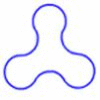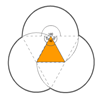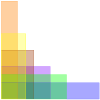# Being Curious - Geometry### Triangle Midpoints

##### Age 14 to 16Challenge Level

You are only given the three midpoints of the sides of a triangle. How can you construct the original triangle?### Blue and White

##### Age 11 to 14Challenge Level

Identical squares of side one unit contain some circles shaded blue. In which of the four examples is the shaded area greatest?### Arclets

##### Age 14 to 16Challenge Level

Each of the following shapes is made from arcs of a circle of radius r. What is the perimeter of a shape with 3, 4, 5 and n "nodes".### Marbles in a Box

##### Age 11 to 16Challenge Level

How many winning lines can you make in a three-dimensional version of noughts and crosses?### Hexy-metry

##### Age 14 to 16Challenge Level

A hexagon, with sides alternately a and b units in length, is inscribed in a circle. How big is the radius of the circle?### Three by One

##### Age 16 to 18Challenge Level

There are many different methods to solve this geometrical problem - how many can you find?### Triangles and Petals

##### Age 14 to 16Challenge Level

An equilateral triangle rotates around regular polygons and produces an outline like a flower. What are the perimeters of the different flowers?### On the Edge

##### Age 11 to 14Challenge Level

If you move the tiles around, can you make squares with different coloured edges?### Sending a Parcel

##### Age 11 to 14Challenge Level

What is the greatest volume you can get for a rectangular (cuboid) parcel if the maximum combined length and girth are 2 metres?### Where to Land

##### Age 14 to 16Challenge Level

Chris is enjoying a swim but needs to get back for lunch. How far along the bank should she land?### Square Coordinates

##### Age 11 to 14Challenge Level

A tilted square is a square with no horizontal sides. Can you devise a general instruction for the construction of a square when you are given just one of its sides?### Right Angles

##### Age 11 to 14Challenge Level

Can you make a right-angled triangle on this peg-board by joining up three points round the edge?### Semi-regular Tessellations

##### Age 11 to 16Challenge Level

Semi-regular tessellations combine two or more different regular polygons to fill the plane. Can you find all the semi-regular tessellations?### Trapezium Four

##### Age 14 to 16Challenge Level

The diagonals of a trapezium divide it into four parts. Can you create a trapezium where three of those parts are equal in area?### Cola Can

##### Age 11 to 14Challenge Level

An aluminium can contains 330 ml of cola. If the can's diameter is 6 cm what is the can's height?### Can They Be Equal?

##### Age 11 to 14Challenge Level

Can you find rectangles where the value of the area is the same as the value of the perimeter?### Cuboid Challenge

##### Age 11 to 16Challenge Level

What's the largest volume of box you can make from a square of paper?### Curvy Areas

##### Age 14 to 16Challenge Level

Have a go at creating these images based on circles. What do you notice about the areas of the different sections?##### Age 14 to 16Challenge Level

Explore when it is possible to construct a circle which just touches all four sides of a quadrilateral.### Which Solids Can We Make?

##### Age 11 to 14Challenge Level

Interior angles can help us to work out which polygons will tessellate. Can we use similar ideas to predict which polygons combine to create semi-regular solids?### Opposite Vertices

##### Age 11 to 14Challenge Level

Can you recreate squares and rhombuses if you are only given a side or a diagonal?### Fit for Photocopying

##### Age 14 to 16Challenge Level

Explore the relationships between different paper sizes.### Vector Journeys

##### Age 14 to 18Challenge Level

Charlie likes to go for walks around a square park, while Alison likes to cut across diagonally. Can you find relationships between the vectors they walk along?### Perimeter Possibilities

##### Age 11 to 14Challenge Level

I'm thinking of a rectangle with an area of 24. What could its perimeter be?